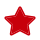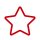# 开发自定义脚本

• 代码目录路径

您需要在OBS桶中指定代码目录，并将训练代码、依赖安装包或者预生成模型等训练所需文件上传至该代码目录下。训练作业创建完成后，ModelArts会将代码目录及其子目录下载至后台容器中。

例如：OBS路径“obs://obs-bucket/training-test/demo-code”作为代码目录，OBS路径下的内容会被自动下载至训练容器的“\${MA_JOB_DIR}/demo-code”目录中，demo-code为OBS存放代码路径的最后一级目录，用户可以根据实际修改。

请注意不要将训练数据放在代码目录路径下。训练数据比较大，训练代码目录在训练作业启动后会下载至后台，可能会有下载失败的风险。建议训练代码目录大小不超过50MB。

• 代码目录路径中的启动文件

代码目录路径中的启动文件作为训练启动的入口，当前只支持python格式。

• 训练输入路径参数

训练数据需上传至OBS桶或者存储至数据集中。在训练代码中，用户需解析输入路径参数。系统后台会自动下载输入参数路径中的训练数据至训练容器的本地目录。请保证您设置的桶路径有读取权限。在训练作业启动后，ModelArts会挂载硬盘至“/cache”目录，用户可以使用此目录来存储临时文件。“/cache”目录大小请参考训练环境中不同规格资源“/cache”目录的大小

• 训练输出路径参数

建议设置一个空目录为训练输出路径。在训练代码中，您需要解析输出路径参数。系统后台会自动上传训练输出至指定的训练输出路径，请保证您设置的桶路径有写入权限和读取权限。

#### （可选）引入依赖

1. 当您使用自定义脚本创建算法的时候，如果您的模型引用了其他依赖，您需要在算法管理 > > 创建算法“代码目录”下放置相应的文件或安装包。

#### 解析输入路径参数、输出路径参数

1. 训练代码中需解析输入路径参数和输出路径参数。ModelArts推荐以下方式实现参数解析。
 ``` 1 2 3 4 5 6 7 8 9 10``` ```import argparse # 创建解析 parser = argparse.ArgumentParser(description='train mnist') # 添加参数 parser.add_argument('--data_url', type=str, default="./Data/mnist.npz", help='path where the dataset is saved') parser.add_argument('--train_url', type=str, default="./Model", help='path where the model is saved') # 解析参数 args = parser.parse_args() ```

完成参数解析后，用户使用“data_url”“train_url”代替算法中数据来源和数据输出所需的路径。

2. 在创建训练作业时，填写输入路径和输出路径。

训练输入选择对应的OBS路径或者数据集路径；训练输出选择对应的OBS路径。

图1 训练输入和输出设置#### 训练代码正文和保存模型

```import os
import argparse
import tensorflow as tf

parser = argparse.ArgumentParser(description='train mnist')
parser.add_argument('--data_url', type=str, default="./Data/mnist.npz", help='path where the dataset is saved')
parser.add_argument('--train_url', type=str, default="./Model", help='path where the model is saved')
args = parser.parse_args()

mnist = tf.keras.datasets.mnist

(x_train, y_train), (x_test, y_test) = mnist.load_data(args.data_url)
x_train, x_test = x_train / 255.0, x_test / 255.0

model = tf.keras.models.Sequential([
tf.keras.layers.Flatten(input_shape=(28, 28)),
tf.keras.layers.Dense(128, activation='relu'),
tf.keras.layers.Dropout(0.2),
tf.keras.layers.Dense(10)
])

loss_fn = tf.keras.losses.SparseCategoricalCrossentropy(from_logits=True)
loss=loss_fn,
metrics=['accuracy'])
model.fit(x_train, y_train, epochs=5)

model.save(os.path.join(args.train_url, 'model'))```

#### 新旧版训练代码适配的差异

```#解析命令行参数
import argparse
parser = argparse.ArgumentParser(description='MindSpore Lenet Example')
help='path where the dataset is saved')
parser.add_argument('--train_url', type=str, default="./Model", help='if is test, must provide\
path where the trained ckpt file')
args = parser.parse_args()
...
#下载数据参数至容器本地，在代码中使用local_data_path代表训练输入位置
mox.file.copy_parallel(args.data_url, local_data_path)
...
#上传容器本地数据至obs路径
mox.file.copy_parallel(local_output_path, args.train_url)```

# 相关产品